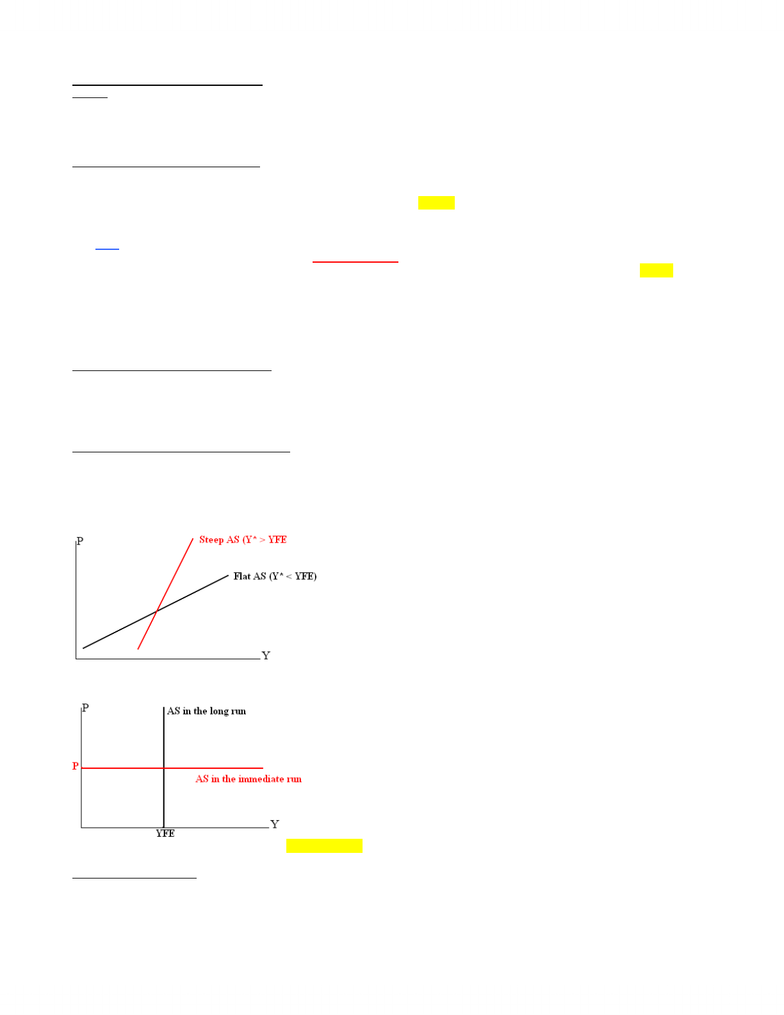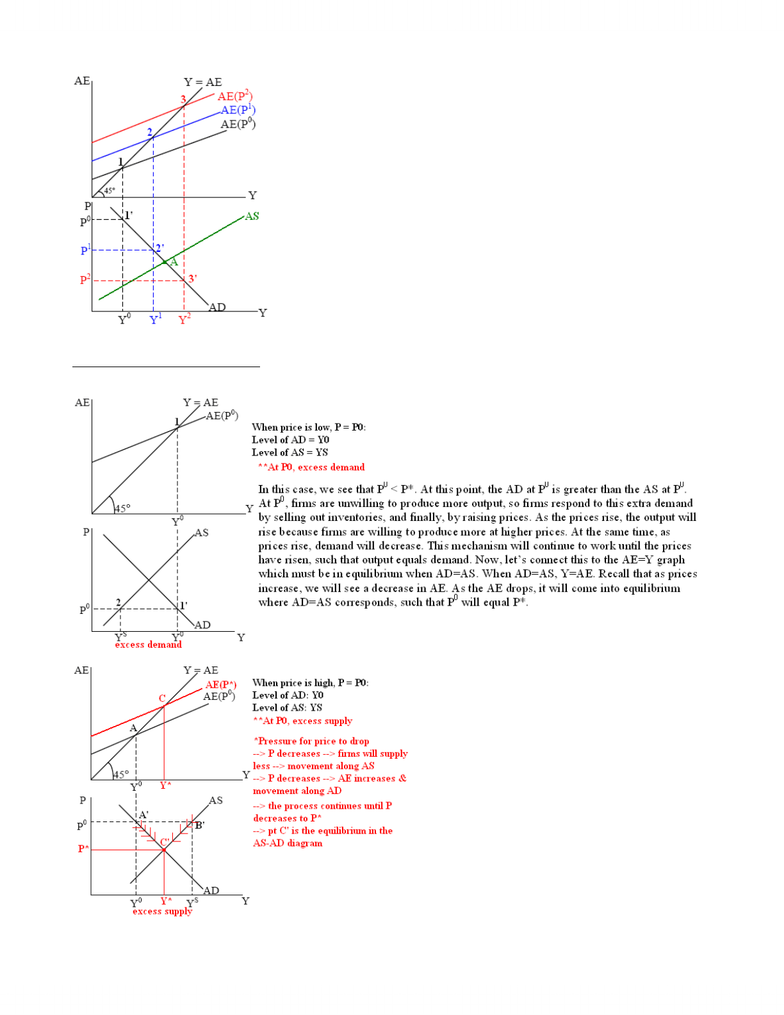# MGEA06H3 Lecture Notes - Lecture 6: Shortage, Aggregate Supply, Marginal Cost

64 views5 pagesOutline
x The derivation of the aggregate supply (AS) curve.
x Consider the effect of a disturbance in the AS-AD model and its implications on government policy.
Derivation of the Aggregate Supply (AS)
x We have already derived the AD curve. We need to derive the aggregate supply curve to complete the model.
o The AD curve indicates the equilibrium level of output on the demand side.
o In other words, the AD curve indicates, at each different price level, the level of output that generates just enough AE (C + I
+ G + X – IM) so that all the output gets purchased (remember we derived the AD curve by setting Y = AE).
x BUT, we need to consider what firms are willing to produce.
o What we have not captured is whether, at that price level, firms will indeed be willing to produce that level of output.
x Therefore, the aggregate supply (AS) curve should represent the amount of output that firms in the economy are willing to
produce at each price level.
x Question: What we learned about marginal cost (MC) in micro suggests that AS should be upward sloping. Does it hold true for the
economy as a whole?
x Answer: Once again we are looking at the entire economy not just one industry, the big picture matters (the level of unemployment
and time frame—short run vs. long run)!
Slope of the AS Curve
Case 1: If There is a Lot of Unemployment
x This means Y* is much lower than YFE (Y* < YFE).
x If this is the case, we might expect that firms will be willing to supply more even if price does not increase much.
o The AS curve will be relatively flat (i.e., elastic). Why?
o Since there are quite a large number of unemployed workers, firms would find it easy to hire workers if they want to expand.
Besides, firms probably have laid off workers in the last little while and have unused facilities.
Case 2: If There is a Not Much Unemployment
x This means Y* is bigger than YFE (Y* > YFE).
x If this is the case, we might expect that firms will be hard-pressed to supply much more even if price increases.
o The AS curve will be relatively steep (i.e., inelastic). Why?
o In order to expand production firms need to hire more workers. However, firms may find it difficult to hire additional
workers, especially good, productive workers. Besides, it may not be feasible to have the existing workers work over time (it
is either too expensive to the firms or the workers may not willing to do so).
x Sometimes, we simply the AS curve as follow:
o A vertical AS curve in the long run (when Y = YFE).
o A horizontal AS curve in the immediate run.
x In the meantime, we assume AS curve is upward sloping—firms are willing to produce more when price rises.
x AD is derived from setting AE to Y at different price levels.
x For the AS curve:
o When the price is low, firms are not willing to produce a lot Æ the level of AS is low.
o When price begins to rise, firms are willing to produce more Æ the level of AS begins to increase.
www.notesolution.com
Unlock document

This preview shows pages 1-2 of the document.
Unlock all 5 pages and 3 million more documents.x When AS intersects AD, the economy is in equilibrium (pt A).
x Question: How do we ensure that the economy ends up at this equilibrium (point A)?
Case 1: Initial Price is Below Equilibrium Price, P0 < P*
Case 2: Initial Price is Above Equilibrium Price, P0 > P*
www.notesolution.com
Unlock document

This preview shows pages 1-2 of the document.
Unlock all 5 pages and 3 million more documents.

## Document Summary

The derivation of the aggregate supply (as) curve. N consider the effect of a disturbance in the as-ad model and its implications on government policy. N we have already derived the ad curve. We need to derive the aggregate supply curve to complete the model. N recap, what do we learn about the ad: the ad curve indicates the equilibrium level of output on the demand side. In other words, the ad curve indicates, at each different price level, the level of output that generates just enough ae (c + i. + g + x im) so that all the output gets purchased (remember we derived the ad curve by setting y = ae). N but, we need to consider what firms are willing to produce: what we have not captured is whether, at that price level, firms will indeed be willing to produce that level of output.

# Get access

\$10 USD/m
Billed \$120 USD annually
Homework Help
Class Notes
Textbook Notes